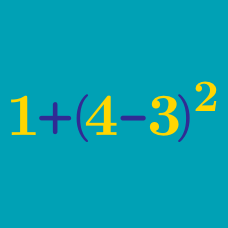Basic Mathematics

# Partition Puzzle Warmup

Arrange the numbers into two sets whose sum is equal.

2, 3, 5, 6

Which of these is in the same set as 2?

Arrange the numbers into two sets so that both sets have the same total sum.

1, 2, 4, 6, 7

Which of these is in the same set as 1?

Arrange the numbers into two sets so that both sets have the same total sum.

2, 3, 5, 5, 6, 7

Which of these is in the same set as 6?

Arrange the numbers into two sets so that both sets have the same total sum.

1, 6, 8, 9, 10, 12

Which of these is in the same set as 10?

Arrange the numbers into two sets so that both sets have the same total sum.

1, 2, 3, 8, 8

Which of these is in the same set as 1?

×

Problem Loading...

Note Loading...

Set Loading...